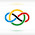### 2007 IMO SL #C7

Let $\alpha < \frac {3 - \sqrt {5}}{2}$ be a positive real number. Prove that there exist positive integers $n$ and $p > \alpha \cdot 2^n$ for which one can select $2 \cdot p$ pairwise distinct subsets $S_1, \ldots, S_p, T_1, \ldots, T_p$ of the set $\{1,2, \ldots, n\}$ such that $S_i \cap T_j \neq \emptyset$ for all $1 \leq i,j \leq p$.

Let $a_0, \dots, a_{k-1}$ be positive integers, and define $s_i=a_0+\cdots+a{i-1}$; let $s_k=s$. Note $a_0=0$. Now, we partition the set $\{1, \dots, s\}$ into segments $I_i = \{s_i + 1, \dots, s_i + a_i\}$ for all $i$. Define two properties on subsets of $\{1, \dots, s\}$, namely that a subset has property $P$ if it contains one of the segments $I_0, \dots, I_{k-1}$, and a subset has property $Q$ if it contains at least one point from each of the segments $I_0, \dots, I_{k-1}$. Note that every subset with property $P$ intersects with every subset with property $Q$.

Next, notice that $2^{-s} |Q| = \prod (1 - 2^{-a_i})$, $2^{-s} |P| = 1 - \prod (1 - 2^{-a_i})$, and $$2^{-s} |P \cap Q| = \prod (1 - 2^{-a_i}) - \prod (1 - 2^{-a_i+1}).$$

Let the elements of $P$ be the $S_i$s and the elements of the set $Q\setminus P$ be the $T_i$s. For the problem condition to hold true, we must have $$\prod (1 - 2^{-a_i}) < 1 - \alpha$$ and  $$\prod (1 - 2^{-a_i+1}) > \alpha.$$

Now, we will consider solutions for $a_0=\cdots=a_{k-1}=a$, where we must have $$(1 - 2^{-a})^k < 1 - \alpha$$ and $$(1 - 2^{-a + 1})^k > \alpha.$$

For brevity, denote $\lambda = \frac{3 - \sqrt{5}}{2}$, and then we have $$\alpha < \lambda = (1 - \lambda)^2 < (1 - \alpha)^2.$$ Pick $k_a = \left \lfloor -2^a \log (1 - \lambda) \right \rfloor$ for all $a$. Then, $$\lim_{k \to \infty} (1 - 2^{-a})^{k_a} = 1 - \lambda$$ and $$\lim_{k \to \infty} (1 - 2^{-a+1})^{k_a} = (1 - \lambda)^2 = \lambda.$$

Finally, we can say that for large $a$, both of the wanted inequalities hold true.

Remark: It can be shown that the condition of $\frac{3-\sqrt{5}}{2}$ is sharp.

1.Anonymous1/15/2022

how so pr0

2.schukkayapally1/15/2022

wao orz

3.schukkayapally1/16/2022

get a fucking custom domain tho!!! when r u gonna get it??

4.@schukkayapally

I appreciate your concern, but please refrain from using bad language and commenting the same thing over and over again in different posts.

I will get a custom domain when I want to.